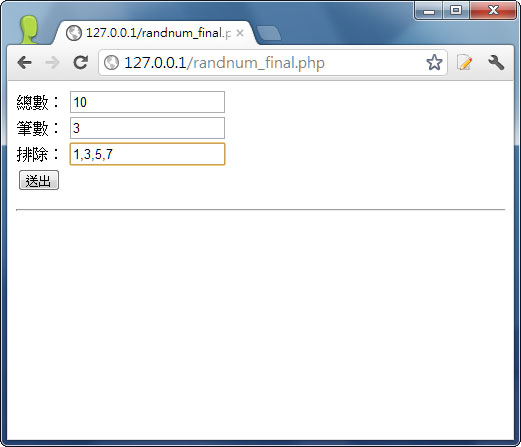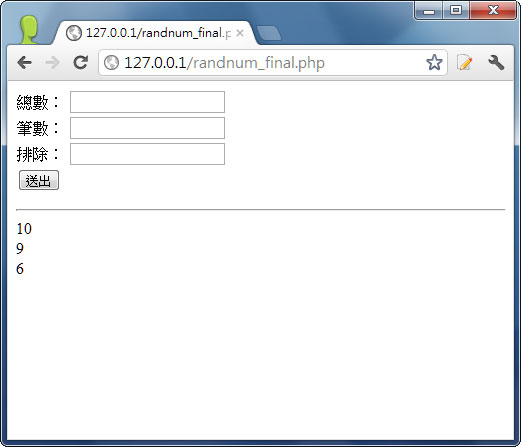1. 首頁
2. »
3. 網頁開發
4. »
5. PHP
6. »
7. PHP教學－亂數隨機抽出得獎者名單

# PHP教學－亂數隨機抽出得獎者名單

2012/03/07先前梅前題曾舉辦了線上抽獎的小活動，也感謝各位網友的熱愛與支持，而每次最令梅干比較感到頭痛的是，當在活動截止日，要抽出得獎者時，就得四處找線上的抽獎的程式，來將幸運的得獎者抽出，由於梅干的記性實在不太好，因此每次都需要花一些時間找尋，所以在上次的活狀抽獎時，梅干就決定自已來寫一個抽獎程式，這樣就不用每次找半天了，並且梅干還加入了排除名單，比方像是資料不全，或是重覆參加者，就可將這些名單排除，再抽出所要的得獎者名單，而梅干也將程式碼整理了一下，並分享給各位，有需要的朋友，直接下載就可使用囉！

index.php

```<form id=“form1” name=“form1” method=“post” action=“”>

<input type=“text” name=“total” id=“total” />
<br />

<input type=“text” name=“count” id=“count” />
<br />

<input name=“except” type=“text” id=“except”  />
<br />
<input type=“submit” name=“button” id=“button” value=“送出” />
</form>
<hr>
<?php
if(isset(\$_POST[‘total’]) && isset(\$_POST[‘count’])){
\$POST_total = \$_POST[‘total’];
\$POST_count = \$_POST[‘count’];
\$POST_except = \$_POST[‘except’];

```\$Rand = Array(); //定義陣列
\$count = \$POST_count ; //共產生幾筆

if(isset(\$_POST['except'])){
/*文字轉陣列－排除名單*/
\$Rand = explode(",",\$POST_except);
}

while(\$count &gt; 0){
\$randval = mt_rand(1,\$POST_total); //取亂數

if (!in_array(\$randval, \$Rand)) {
\$count--;
\$Rand[] = \$randval; //若無重復則 將亂數塞入陣列
echo \$randval."&lt;br&gt;";
}
}
```

}
?>
```

#1#2哈～其實程式碼沒幾行，有興趣的朋友，也可自行下載研究，沒興趣的朋友，下載後就可直接使用了，而這次也要感謝好友男丁的協助，幫梅干進行一些除錯，讓這隻程式更加完整。

[範例下載]